### APPENDIX IV. NUMERICAL METHODS FOR MAXIMUM LIKELIHOOD AND LEAST SQUARES SOLUTIONS

In many cases the likelihood function is not analytical or else, if analytical, the procedure for finding thej* and the errors is too cumbersome and time consuming compared to numerical methods using modern computers.

For reasons of clarity we shall first discuss an inefficient, cumbersome method called the grid method. After such an introduction we shall be equipped to go on to a more efficient and practical method called the method of steepest descent.

The grid method

If there are M parameters1, ... ,M to be determined one could in principle map out a fine grid in M-dimensional space evaluating w() (or S()) at each point. The maximum value obtained for w is the maximum likelihood solution w*. One could then map out contour surfaces of w = (w* - ½), (w* - 1), etc. This is illustrated for M = 2 in Fig. 6.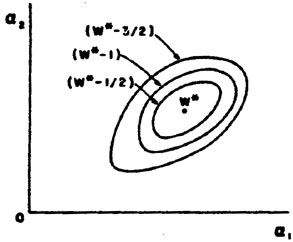Figure 6. Contours of fixed w enclosing the max. likelihood solution w*.

In the case of good statistics the contours would be small ellipsoids. Fig. 7 illustrates a case of poor statistics.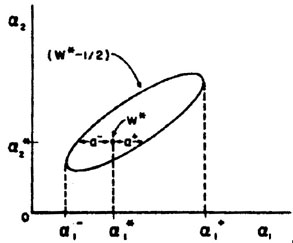Figure 7. A poor statistics case of Fig. 6.

In the case of good statistics the second derivatives ð2w / ða ðb = - Hab could be found numerically in the region near w*. The errors in the's are then found by inverting the H-matrix to obtain the error matrix for; i.e.,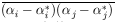= (H-1)ij. The second derivatives can be found numerically by usingIn the case of least squares use Hij = ½ ðS / ði ðj .

So far we have for the sake of simplicity talked in terms of evaluating w() over a fine grid in M-dimensional space. In most cases this would be much too time consuming. A rather extensive methodology has been developed for finding maxima or minima numerically. In this appendix we shall outline just one such approach called the method of steepest descent. We shall show how to find the least squares minimum of S(). (This is the same as finding a maximum in w()).

Method of Steepest Descent

At first thought one might be tempted to vary1 (keeping the other's fixed) until a minimum is found. Then vary2 (keeping the others fixed) until a mew minimum is found, and so on. This is illustrated in Fig. 8 where M = 2 and the errors are strongly correlated. But in Fig. 8 many trials are needed. This stepwise procedure does converge, but in the case of Fig. 8, much too slowly. In the method of steepest descent one moves against the gradient in-space: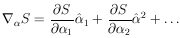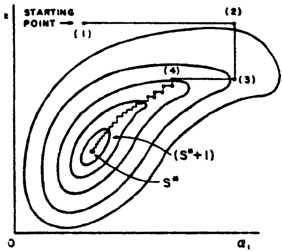Figure 8. Contours of constant S vs.1 and2. Stepwise search for the minimum.

So we change all the's simultaneously in the ratio ðS / ð1 : ðS / ð2 : ðS / ð3 : ... . In order to find the minimum along this line in-space one should use an efficient step size. An effective method is to assume S(s) varies quadratically from the minimum position s* where s is the distance along this line. Then the step size to the minimum is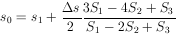where S1, S2, and S3 are equally spaced evaluations of S(s) along s with step sizes starting from s1; i.e., s2 = s1 +s, s3 = s1 + 2s. One or two iterations using the above formula will reach the minimum along s shown as point (2) in Fig. 9. The next repetition of the above procedure takes us to point (3) in Fig. 9. It is clear by comparing Fig. 9 with Fig. 8 that the method of steepest descent requires much fewer computer evaluations of S() than does the one variable at a time method.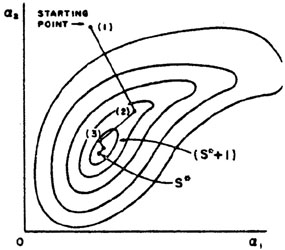Figure 9. Same as Fig. 8, but using the method of steepest descent.

Least Squares with Constraints

In some problems the possible values of thej are restricted by subsidiary constraint relations. For example, consider an elastic scattering event in a bubble chamber where the measurements yj are track coordinates and thei are track directions and momenta. However, the combinations ofi that are physically possible are restricted by energy-momentum conservation. The most common way of handling this situation is to use the 4 constraint equations to eliminate 4 of the's in S(). Then S is minimized with respect to the remaining's. In this example there would be (9 - 4) = 5 independent's: two for orientation of the scattering plane, one for direction of incoming track in this plane, one for momentum of incoming track, and one for scattering angle. There could also be constraint relations among the measurable quantities yi. In either case, if the method of substitution is too cumbersome, one can use the method of Lagrange multipliers.

In some cases the constraining relations are inequalities rather than equations. For example, suppose it is known that1 must be a positive quantity. Then one could define a new set of's where (1')2 =1,2' =2, etc. Now if S(') is minimized no non-physical values of a will be used in the search for the minimum.